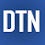## Pages

### Regression Example with SGDRegressor in Python

Applying the Stochastic Gradient Descent (SGD) method to the linear classifier or regressor provides the efficient estimator for classification and regression problems.

Scikit-learn API provides the SGDRegressor class to implement SGD method for regression problems. The SGD regressor applies regularized linear model with SGD learning to build an estimator. A regularizer is a penalty (L1, L2, or Elastic Net) added to the loss function to shrink the model parameters. The SGD regressor works well with large-scale datasets.

In previous post, we learned how to classify data with SGD classifier in Python and you can find it here.

In this tutorial, we'll briefly learn how to fit and predict regression data by using Scikit-learn's SGDRegressor class in Python. The tutorial covers:
1. Preparing the data
2. Training the model
3. Predicting and accuracy check
4. Boston dataset prediction
5. Source code listing

```from sklearn.linear_model import SGDRegressor
from sklearn.datasets import make_regression
from sklearn.metrics import mean_squared_error
from sklearn.model_selection import train_test_split
from sklearn.model_selection import cross_val_score
from sklearn.preprocessing import scale
import matplotlib.pyplot as plt ```
```
```

Preparing the data

First, we'll generate random regression data with make_regression() function. The dataset contains 30 features and 1000 samples.

```x, y = make_regression(n_samples=1000, n_features=30)

```

To improve the model accuracy we'll scale both x and y data then, split them into train and test parts. Here, we'll extract 15 percent of the samples as test data.

```x = scale(x)
y = scale(y)xtrain, xtest, ytrain, ytest=train_test_split(x, y, test_size=0.15)
```

Training the model

Next, we'll define the regressor model by using the SGDRegressor class. Here, we can use default parameters of the SGDRegressor class.

```sgdr = SGDRegressor()
print(sgdr)```

Then, we'll fit the model on train data and check the model accuracy score.

```sgdr.fit(xtrain, ytrain)

score = sgdr.score(xtrain, ytrain)
print("R-squared:", score)
R-squared: 0.9999999253180197```
` `

We can also apply a cross-validation method to the model and check the training accuracy.

```cv_score = cross_val_score(sgdr, x, y, cv = 10)
print("CV mean score: ", cv_score.mean())
CV mean score:  0.9999999624822019```

Predicting and accuracy check

Now, we can predict the test data by using the trained model. We can check the accuracy of predicted data by using MSE and RMSE metrics.

```ypred = sgdr.predict(xtest)

mse = mean_squared_error(ytest, ypred)
print("MSE: ", mse)
print("RMSE: ", mse**(1/2.0)) ```
` `
`MSE:  1.1979434697284535e-07RMSE:  5.989717348642267e-08 `
` `

Finally, we'll visualize the original and predicted data in a plot.

```x_ax = range(len(ytest))
plt.plot(x_ax, ytest, linewidth=1, label="original")
plt.plot(x_ax, ypred, linewidth=1.1, label="predicted")
plt.title("y-test and y-predicted data")
plt.xlabel('X-axis')
plt.ylabel('Y-axis')
plt.grid(True)
plt.show() ```

Boston housing dataset prediction

We'll apply the same method we've learned above to the Boston housing price regression dataset. We'll load it by using load_boston() function, scale and split into train and test parts. Then, we'll define model by changing some of the parameter values, check training accuracy, and predict test data.

```print("Boston housing dataset prediction.")
x, y = boston.data, boston.target

x = scale(x)
y = scale(y)
xtrain, xtest, ytrain, ytest = train_test_split(x, y, test_size=.15)

sgdr = SGDRegressor(alpha=0.0001, epsilon=0.01, eta0=0.1,penalty='elasticnet')
sgdr.fit(xtrain, ytrain)

score = sgdr.score(xtrain, ytrain)
print("R-squared:", score)

# cv_score = cross_val_score(lsvr, x, y, cv=5)
# print("CV mean score: ", cv_score.mean())

ypred = sgdr.predict(xtest)

mse = mean_squared_error(ytest, ypred)
print("MSE: ", mse)
print("RMSE: ", mse**(1/2.0))

x_ax = range(len(ytest))
plt.plot(x_ax, ytest, label="original")
plt.plot(x_ax, ypred, label="predicted")
plt.title("Boston test and predicted data")
plt.xlabel('X-axis')
plt.ylabel('Y-axis')
plt.grid(True)
plt.show()```
` `
`Boston housing dataset prediction.R-squared: 0.7239074887406243MSE:  0.2200176437469965RMSE:  0.11000882187349825 `
` `

In this tutorial, we've briefly learned how to fit and predict regression data by using Scikit-learn API's SGDRegressor class in Python. The full source code is listed below.

Source code listing

` `
```from sklearn.linear_model import SGDRegressor
from sklearn.datasets import make_regression
from sklearn.metrics import mean_squared_error
from sklearn.model_selection import train_test_split
from sklearn.model_selection import cross_val_score
from sklearn.preprocessing import scale
import matplotlib.pyplot as plt

x, y = make_regression(n_samples=1000, n_features=30)
print(x[0:2])
print(y[0:2])

x = scale(x)
y = scale(y)
xtrain, xtest, ytrain, ytest = train_test_split(x, y, test_size=.15)

sgdr = SGDRegressor()
print(sgdr)

sgdr.fit(xtrain, ytrain)

score = sgdr.score(xtrain, ytrain)
print("R-squared:", score)

cv_score = cross_val_score(sgdr, x, y, cv=10)
print("CV mean score: ", cv_score.mean())

ypred = sgdr.predict(xtest)

mse = mean_squared_error(ytest, ypred)
print("MSE: ", mse)
print("RMSE: ", mse**(1/2.0))

x_ax = range(len(ytest))
plt.plot(x_ax, ytest, linewidth=1, label="original")
plt.plot(x_ax, ypred, linewidth=1.1, label="predicted")
plt.title("y-test and y-predicted data")
plt.xlabel('X-axis')
plt.ylabel('Y-axis')
plt.grid(True)
plt.show()

print("Boston housing dataset prediction.")
x, y = boston.data, boston.target

x = scale(x)
y = scale(y)
xtrain, xtest, ytrain, ytest = train_test_split(x, y, test_size=.15)

sgdr = SGDRegressor(alpha=0.0001, epsilon=0.01, eta0=0.1,penalty='elasticnet')
sgdr.fit(xtrain, ytrain)

score = sgdr.score(xtrain, ytrain)
print("R-squared:", score)

ypred = sgdr.predict(xtest)

mse = mean_squared_error(ytest, ypred)
print("MSE: ", mse)
print("RMSE: ", mse**(1/2.0))

x_ax = range(len(ytest))
plt.plot(x_ax, ytest, label="original")
plt.plot(x_ax, ypred, label="predicted")
plt.title("Boston test and predicted data")
plt.xlabel('X-axis')
plt.ylabel('Y-axis')
plt.grid(True)
plt.show() ```
` `

References:

1.are you missing an asterisk? `print("RMSE: ", mse*(1/2.0))`

1.Yes, and corrected it.

2.What is nsvr? It is referenced calling the predict method, I'm assuming it's supposed to be sgdr.

1.yes, it is sgdr

3.Hello, Thanks for this amazing tutorial! I've a question: How we can deal with one-hot encoded features? should we scale them too?

4.I wanted to get the coefficients as well as bias of the model. I did the following

model = SGDRegressor(random_state=0)
model.fit(x_train, y_train.ravel())
print('coef are ',sgd_fit.coef_)

print('bias is',sgd_fit.intercept_)

1.print("coef are", model.coef_)

5.model = SGDRegressor(random_state=0)
model.fit(x_train, y_train.ravel())
# print(sgd_fit)
#print(sgd_fit.predict(np.zeros((1,8))))
print('coef are ',sgd_fit.coef_)

print('bias is',sgd_fit.intercept_)